# Extension of domain, principle of

Carleman's principle

The harmonic measureof an arcof the boundaryof a domaincan only increase whenis extended across arcs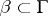,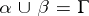. More precisely, let the boundaryof a domainin the complex-plane consist of a finite number of Jordan curves, letbe a part ofconsisting of a finite number of arcs of, and letbe an extension of the domainacross the complementary arcs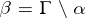, that is,andis a part of the boundary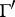of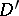. Then for the harmonic measures one has the inequality,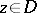, where equality only holds if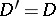. The principle of extension of domain also holds for harmonic measure on domains in the Euclidean space,, or,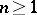.

The principle of extension of domain finds important applications in various problems concerned with estimating harmonic measure. For example, T. Carleman  used the principle of extension of domain to obtain the solution of the Carleman–Milloux problem: Let the boundaryof a simply-connected domainconsist of a finite number of Jordan arcs, let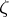be a point on, or let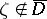, letbe the disc of radiuswith centre, and letbe the part ofin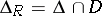. It is required to find a lower bound for the harmonic measure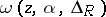depending only onand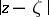,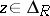. The solution is given by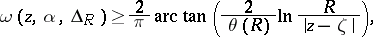(1)

where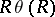is the sum of the lengths of arcs of the intersectionSince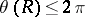, it follows that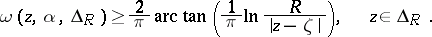(2)

There exist generalizations of the Carleman–Milloux problem and refinements of formulas (1), (2) (see ). The principle of extension of domain also allows one to prove the Lindelöf theorems (cf. Lindelöf theorem). Various applications of the principle of extension of domain and of formulas of the type (1), (2) were given by H. Milloux (see , and also , ).